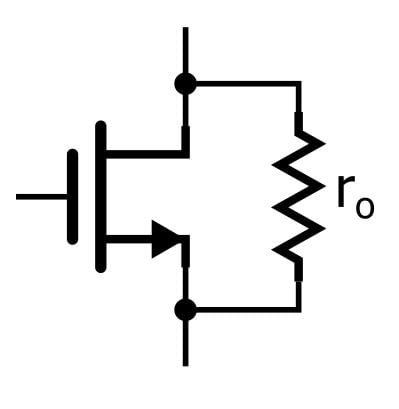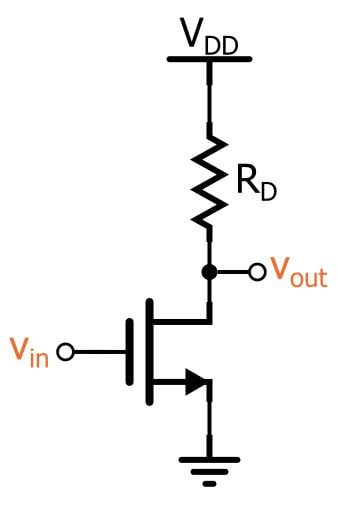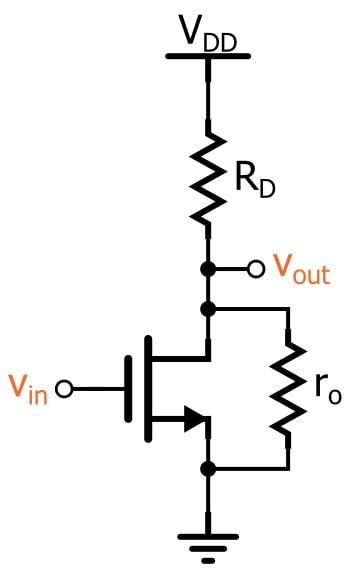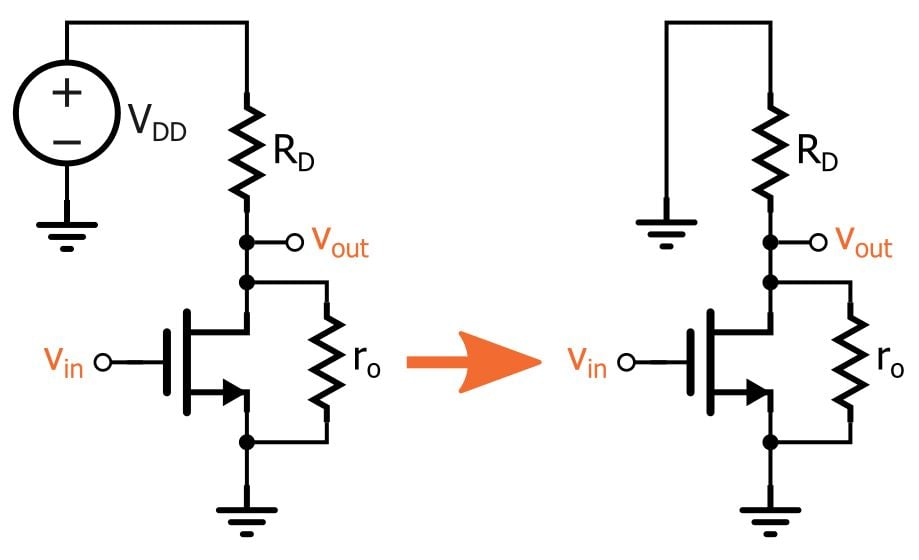Technical Article

# The Actively Loaded MOSFET Differential Pair: Output Resistance

July 05, 2016 by Robert Keim

## In this article, we’ll discuss MOSFET small-signal output resistance as we make our way toward predicting the gain of the actively loaded differential pair.

In this article, we’ll discuss MOSFET small-signal output resistance as we make our way toward predicting the gain of the actively loaded differential pair.

### The Effect of Channel-Length Modulation

In the two previous articles, we introduced the actively loaded MOSFET differential pair and discussed two prominent advantages of this configuration—namely, improved biasing (compared to the use of drain resistors) and differential-to-single-ended conversion without loss of gain.

Now it’s time to analyze the differential gain of this circuit; before we can determine the gain, though, we need to understand the concept of small-signal output resistance and how we incorporate this resistance into our analysis. (If you’re not sure what I mean by “small-signal,” take a look at the “Two Outputs or One?” section in this article.)

The first thing to understand is that small-signal output resistance is not an inherent, precise property of a real MOSFET. Rather, it is a model that we use to account for the effect of channel-length modulation on a MOSFET’s small-signal behavior. Recall that MOSFETs used for linear amplification are typically biased in the saturation region, which corresponds to when the FET’s channel is “pinched off” at the drain end.In a simplified analysis, we use the following equation for saturation-mode drain current:

$$I_D=\frac{1}{2}\mu_nC_{ox}\frac{W}{L}(V_{GS}-V_{TH})^2$$

This equation conveys the assumption that drain current is not affected by the drain-to-source voltage. The MOSFET acts like a dependent current source controlled by the overdrive voltage VOV, where VOV = VGS – VTH. This assumption is based on the idea that increasing the drain-to-source voltage does not alter the channel once it has become pinched off.

As you’ve probably noticed, though, the real world is not particularly conducive to idealized situations such as this.

The reality is that increasing the drain-to-source voltage does have a nontrivial effect on the channel: the pinch-off point is moved toward the source, and the result is more drain-to-source current as drain-to-source voltage increases. This means that we need an additional circuit element to account for this additional current, and by now you have probably guessed that the element we’re looking for is a resistor—namely, the small-signal output resistance ro.So now we have a MOSFET, which is still assumed to be immune to increasing drain-to-source voltage, in conjunction with an ordinary resistor, which (like any resistor) has a current flow equal to the voltage across the resistor divided by the resistance. As drain-to-source voltage increases, more current flows through the resistor, and this current compensates for the lack of change in the drain current of the idealized MOSFET. By combining these two currents—drain current of the idealized FET and current through the resistor—we can find the total drain current for a real MOSFET.

Ignoring channel-length modulation is equivalent to assuming that the small-signal output resistance of the FET is infinite. It follows, then, that higher output resistance is desirable if we want a MOSFET to behave more like the idealized component in which drain current is not influenced by drain-to-source voltage. As we will see later, small-signal output resistance is determined in part by the FET’s DC bias current, so we do have some ability to increase the output resistance of a given device.

One last note before we move on: Output resistance is itself a simplification of real MOSFET behavior. The subatomic action taking place in a MOSFET’s channel is not exactly straightforward, and it comes as no surprise to me that the simple linear relationship represented by a drain-to-source resistor is not the whole story.

### Reducing Gain

How does finite small-signal output resistance affect the performance of a MOSFET amplifier? Consider the following circuit:This is a basic common-source amplifier. We’re concerned only with small-signal behavior here, which means that 1) the biasing circuitry is omitted and 2) you can assume that the FET is in saturation. As discussed in the first section of The MOSFET Differential Pair with Active Load, the magnitude of this amplifier’s gain is the MOSFET’s transconductance multiplied by the drain resistance:

$$A_V=g_m\times R_D$$

Now let’s incorporate the finite output resistance:And next we recall that the small-signal analysis technique allows us to replace constant DC voltage sources with short circuits. The result is the following:Now the effect of the output resistance is clear—it is in parallel with the drain resistor, and thus the magnitude of the voltage gain becomes the following:

$$A_V=g_m\times \left(R_D\parallel r_o\right)$$

So the finite output resistance lowers the gain, because the equivalent resistance of two resistors in parallel is always less than that of either individual resistor. This demonstrates the desirability of higher small-signal output resistance: if ro is much larger than RD, the reduction in gain will be negligible. Notice also that the output resistance places an upper limit on AV; no matter how much drain resistance you have, the equivalent resistance RD||ro will never be higher than ro. This means that AV cannot exceed (gm × ro), which is referred to as a MOSFET’s intrinsic gain.

### Lambda

OK, so how do we calculate the small-signal output resistance? You need two things: bias current and lamba (or λ, for those who are a little foggy on the Greek alphabet).

$$r_o=\frac{1}{\lambda\times I_D}$$

Lambda depends on physical characteristics of the FET and on the device’s bias conditions, but to make life tolerably simple we ignore the dependence on bias conditions and assume that lambda is a constant for a particular process technology.

You should be aware, though, that lambda increases as the length of the physical channel decreases. ID (fortunately more straightforward than lambda) is the FET’s DC-bias drain current—i.e., the drain current that you ignore when performing small-signal analysis.

I looked through some NMOS SPICE models and saw lambda values in the range of, say, 0.01 to 0.1 V–1. With a bias current of 500 µA, this range corresponds to small-signal output resistance of 200 kΩ to 20 kΩ.

This gives you an idea of the high gains we can achieve by loading an amplifier with a transistor’s small-signal output resistance instead of an ordinary drain resistor (if you find this statement confusing, refer back to “The Drain-Resistor Problem” and “Thinking About a Current Source” in this article). Keep in mind, though, that state-of-the-art short-channel MOSFETs will have higher lambda (and thus lower gain).

### Conclusion

Now that we have explored small-signal output resistance, we are ready to analyze the differential gain of our actively loaded MOSFET differential pair. We’ll do this in the next article, and we’ll also empirically (i.e., via simulation) measure lambda so that we can predict the gain of an LTspice differential amplifier.

Next Article in Series: